Courses

Sample GATE Mock Test - Computer Science And IT (CS/IT)

65 Questions MCQ Test GATE Computer Science Engineering(CSE) 2022 Mock Test Series | Sample GATE Mock Test - Computer Science And IT (CS/IT)

Description
Attempt Sample GATE Mock Test - Computer Science And IT (CS/IT) | 65 questions in 180 minutes | Mock test for GATE preparation | Free important questions MCQ to study GATE Computer Science Engineering(CSE) 2022 Mock Test Series for GATE Exam | Download free PDF with solutions
QUESTION: 1

Solution:

Past Tense is used

QUESTION: 2

A rectangular room having dimensions 3.74 m x 5.78 m is required to be tiled using minimum number of identical square tiles. The number of such tiles and area of each tile (in cm2) is given by;

Solution:

In this question, we need to find the HCF of 374 and 578 which is 34. We can look at 374 = 34 x 11 and 578 = 34 x 17. This gives the number of square tiles required to cover the entire room is 11 x 17 = 187 and area of each such tile is 342 = 1156 cm2.

QUESTION: 3

In the following sentence certain parts are underlined and marked P, Q and R. One of the parts may contain certain error or may not be acceptable in standard written communication. Select the part containing an error. Choose D as your answer if there is no error. The student corrected all the errors (P) that the instructor marked (Q) on the answer book (R)

Solution:

In Q part, 'the' is not required.

QUESTION: 4

4 taps marked as T1, T2, T3 and T4 can fill a tank in 8 hours,12 hours, 16 hours and 24hours respectively. We have to fill up 2 identical tanks with 2 out of these 4 taps connected to tank 1 and remaining two taps connected to tank 2 so that the ratio of time taken to fill tank 1 and tank 2 is 2:3. so identify one of the pair of taps?

Solution:

Let us assume the capacity of the tank to be 48 units (48 being LCM of 8, 12, 16 and 24). This leads us to get the rate of filing of T1 to 4 as 6, 4, 3 and 2 units per hour.

Taking pairs of taps, we find that T1 + T2 will take 48/10 whereas the remaining pair will take 48/5 hours leading to the ratio of time taken to fill up 2 tanks as 5:10 which is not the required ratio.

Taking T1 and T3, the time taken is 48/9 and the time taken for the other tank is 48/6 leading to the required ratio as 2:3.

QUESTION: 5

Given below is information related to installed capacity and generation of electricity in India for a specific year: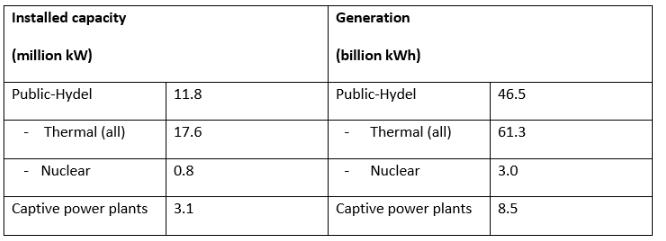The closet integral value of capacity utilization for the entire country in the year under consideration is ____%.

[Capacity utilization is the ratio of Electricity generated per hour and installed capacity. Assume that all plants work 365 days a year for 24 hours per day].

Solution:

Total installed capacity = 33.3 million kW and total power generated = 119.3 billion kWh

Percentage utilization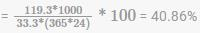which gives nearest integer value as 41%.

QUESTION: 6

12 years ago, age of a father was 4 times of that of his son. 6 years later age of father would be twice of that of son then what is present age of son?

Solution:

Let the ages of father and son 12 years ago were F and S respectively. Then A/Q,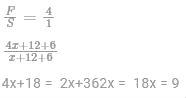Present age of son  x+ 12 = 9+12 = 21 yesrs

QUESTION: 7

A water tank of capacity 6000 liters is connected to 2 taps ‘A’ and ‘B’. Water flows from these 2 taps at 90 liters per minute and 60 liters per minute respectively. To fill this empty tank, first tap ‘A’ is opened for some time and once it is closed, tap ‘B’ is opened till the tank is full taking a total of 90 minutes. What is the difference in the time (in minutes) for which the taps are opened to fill the tank?

Solution:

Let x and y be the time for which tap ‘A’ and ‘B’ are opened

We have x + y = 90 … (i)

90x + 60y = 6000 … (ii)

Solving (i) and (ii), gives x = 20 and y = 70.

⇒  Difference in the duration for which taps ‘A' and ‘B’ are opened = 70 – 20 = 50 minutes.

QUESTION: 8

Two trains from Delhi to Ranchi and from Ranchi to Delhi started at the same time. Due to fog in winter season, after crossing each other, they took 16 hr and 9 hr respectively to complete their journey. If the speed of train going to Ranchi was 45 km/h, what was the speed of train going to Delhi?

Solution:

Let them meet at X after t hours and speed of train 2 be s kmph as shown in figure below

Train 1 is shown above the line and Train 2 is shown below the line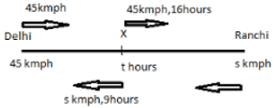Distance travelled by train 1 before meeting = Distance travelled by train 2 after meeting

45*t = 9*s   .... i

Also Distance travelled by train 2 before meeting = Distance travelled by train 1 after meeting

st = 45*16 .... ii

Using eq i ;

t= s/5

Putting in eq ii ;

s^2 / 5 = 45*16

s=60 kmph

OR

Short trick:

Let the speed of train going from Ranchi to Delhi = u km/h

And speed of train going from Delhi to Ranchi = v km/h

Then,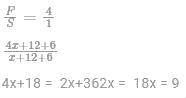QUESTION: 9

Chinnaswamy is driving to pick up his son from school on a Saturday which is a half day. On his way to school, he crossed a church which is 1/5th of the way to school at 9:50 hours and exactly 10 minutes later, he went past a temple which is 1/3rd of the way to school. The time after 10:00 hours at which he reaches his son’s school is ____ minutes.

Solution:

Since we are given time to cross a point which is 1/5th of the way and 1/3rd of the way, we can rewrite them as 3/15th and 5/15th of the way which implies that 2/15th of the way was covered in 10 minutes.

This means that the entire route was covered in 10 × 15/2 = 75 minutes (each 1/15th part is covered in 75/15 = 5 minutes) leading to time of arrival at school as 9:50 – 15 + 75 = 10: 50 hours.

QUESTION: 10

A group of 3000 students, which includes 1750 girls, in a school are engaged in exactly one of the 5 activities as per details given below in the table. What is the difference in the number of boys opting for craft and dancing when compared with drawing and swimming?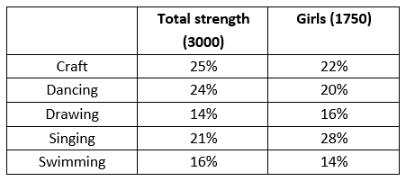Solution: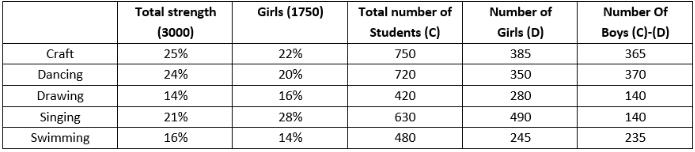Total number of boys opting for craft and dancing = 365 + 370 = 735

Total number of boys opting for drawing and swimming = 140 + 235 = 375

Required difference = 735 - 375 =  360

QUESTION: 11

Which of the following is not the disc scheduling algorithm.

Solution:

In operating systems, seek time is very important. Since all device requests are linked in queues, the seek time is increased causing the system to slow down. Disk Scheduling Algorithms are used to reduce the total seek time of any request.

TYPES OF DISK SCHEDULING ALGORITHMS

Although there are other algorithms that reduce the seek time of all requests, I will only concentrate on the following disk scheduling algorithms:

First Come-First Serve (FCFS)

Shortest Seek Time First (SSTF)

Elevator (SCAN)

Circular SCAN (C-SCAN)

LOOK

C-LOOK.

QUESTION: 12

For slow and inefficient I/O peripherals, the interrupt mechanism to be designed must be fast enough. The best possible choice for interrupt handling would be;

Solution:

Vectored interrupts are the fastest. Slow and inefficient peripherals are not able to provide device identification number or ISR address. Best option is to have ISR at a fixed address. So for a n-bit CPU, ISR of peripheral attached to vector x will be at x*8.

QUESTION: 13

What is the time complexity to construct binary search tree when inorder and postorder traversal of tree is given?

Solution:

For each node in preorder linear search in the order traversal gives left subtree and right subtree.

Time complexity =O(n2)

QUESTION: 14

The different bus arbitration techniques are daisy chaining, polling and independent requesting. The bus grant and bus request lines are only assumed as control lines. For n requesting devices, the number of control lines for daisy chaining, polling and independent requesting are respectively;

Solution:

For daisy chaining, there is a single bus request line and bus grant line which is passed through each of the devices. In polling, there is log2(n) bit polling number for the sequence maintaining I/O devices. Bus is granted using a poll count. Thus log2(n) lines needed to maintain poll count. Bus request line is single. So total no of control lines = log2(n)+1. For independent requesting separate bus request and bus grant line for each I/o device. Thus 2n control lines needed.

QUESTION: 15

Consider a system has three processes and three resources are available of same type. If each process needs maximum two resources, which of the following is correct?

Solution:

Deadlock occur if each and every process holds one resource and request resource of other process such that circular wait condition get satisfied. Let A, B, C be these processes holding one process each.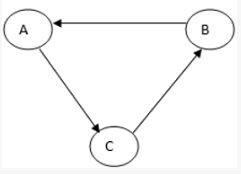*Answer can only contain numeric values
QUESTION: 16

Assume that source S and destination D are connected through two routers. Also there exists a gateway between these two routers. Let 'x' be the number of times a packet visits transport layer, 'y' be the number of times a packet visits network layer and 'z' be the number of times a packet visits data link layer during transmission from source to destination. Then calculate x+2y+3z = __________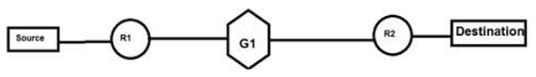Solution:

At source , packet visits all the layer once before getting transmitted.

At router R1 , it will go upto the network layer because router is a networking device.

At gateway G1 , it will go upto transport layer because gateway is an all layer switch who works on transport layer.

At router R2 , it will go upto network layer.

At destination , packet will once again visit all the layers.

Hence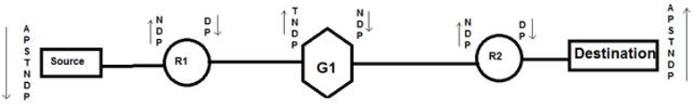A - Application Layer

P - Presentation Layer

S - Session Layer

T - Transport Layer

N - Network Layer

P - Physical Layer

Hence , from figure

x = 3 y= 6 z = 8

so, x + 2y + 3z = 3 + 12 + 24 = 39

QUESTION: 17

A student want to prove a relation between the “gradeup” and “gaterank”. If he prove that gradeup is reducible to “gaterank” and “gaterank” is decidable then which of the following is true? (Assume gradeup & gaterank are two problems)

Solution:

gaterank is decidable.

If gaterank is decidable, then gradeup is also decidable.

So option (a) is correct.

QUESTION: 18

Which of the following is functionality complete set?

Solution:

NOT and OR can implement NOR (universal gate). Therfore {NOT, OR} is functionally complete. NOR and NAND are designated as universal logic gates because using any one of them we can implement all the logic gates.

QUESTION: 19

Consider the following dependencies in a database

D → A A → E

N → R R → N

C →  C → I

(R, C) → G

The relation (R, N, D, A) is

Solution:

D →A A → E

N →R R → N

C →  C →I

(R, C) →G

From the given relation

R( R, N, D, A)

The functional dependencies which this particular relation hold:

D →A N →R R → N

The candidate key for the relation will be: RD, DN

Hence partial dependency exists in the given relation R:

D →A

So relation is in 1NF but not in 2NF.

QUESTION: 20

If L1 is DCFL and L2 is CFL , then which statement is true:

Solution: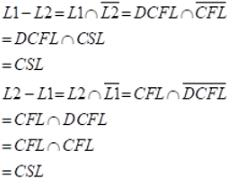*Answer can only contain numeric values
QUESTION: 21

Consider the matrix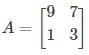Find the eigenvalue of A for which the normalized eigen vector is given by;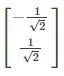Solution: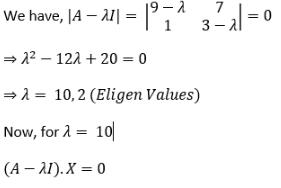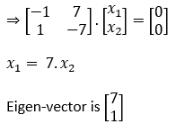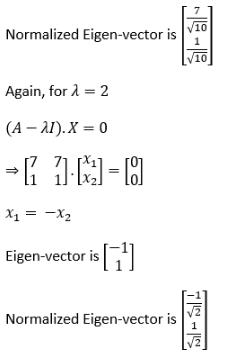QUESTION: 22

Consider the three problems :

• Equivalence
• Ambiguity
• Regularity
Solution:

For regular language

1) Equivalence: it can be checked by developed product automate for XOR function.

if XOR = O ⇒ equal else not.

2) Ambiguity: Ambiguity is decidable for regular languages & grammar since they are deterministic in nature.

3) Regularity: it is the trivial problem.

For context free language

⇒ it is not closed in any of those problems.

QUESTION: 23

For the matrix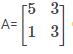ONE of the normalized eigen vector is given as;

Solution: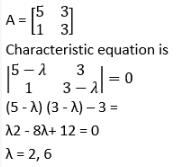Now, to fine eigen vectors;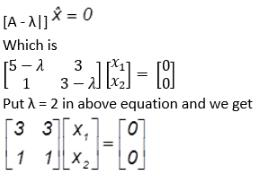which give us the equation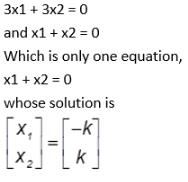so one eigen vector is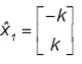which after normalization is =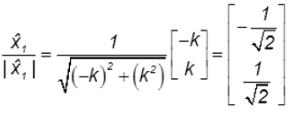the other eigen vector is obtained by putting the other eigen value λ = 6 I eigen value problem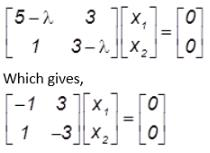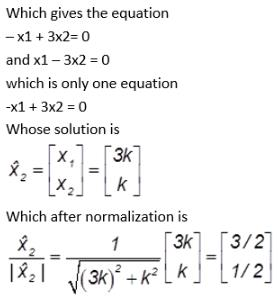choice B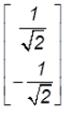is the only correct choice ,

since it is a constant multiple of one the normalized vector which is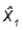QUESTION: 24

IPv4 addressing system already gives information about network and host. Along with the Internet Protocol address of host, subnet mask is also needed by the routers inorder to direct the packet to intended network. Host doesn’t redirect packets, then Why does host need subnet mask?

Solution:

We know Subnet mask is needed by routers (stored in routing table) inorder to direct the packet to intended network. But how will host know whether the packet belongs to its own network, whether to send the packet to router or not. For this reason, even host needs the Subnet mask.

QUESTION: 25

Consider the following BST.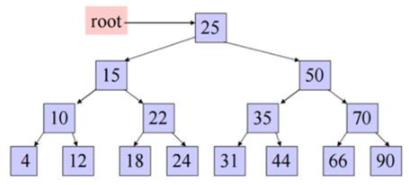Its pre-order and post-order are inserted in two separate arrays(arrays having same starting index) of same size in traversal sequence. How many elements will have same index numbers in the both the arrays?

Solution:

A Pre-order traversal visits nodes in the following order:

25, 15, 10, 4, 12, 22, 18, 24, 50, 35, 31, 44, 70, 66, 90

A Post-order traversal visits nodes in the following order:

4, 12, 10, 18, 24, 22, 15, 31, 44, 35, 66, 90, 70, 50, 25

Hence 4 elements are having same index numbers in both the arrays.

QUESTION: 26

Consider two relations R1 and R2 given below: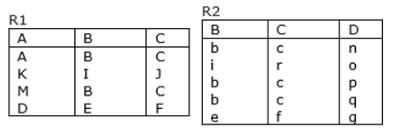How many tuples are there in relation (Natural join) R1 R2?

Solution:

Explanation: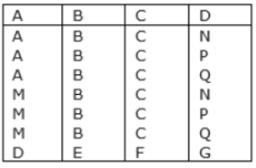QUESTION: 27

In the spanning tree shown, what will be the minimum cost?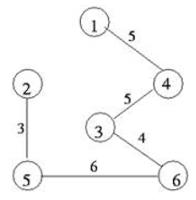Solution:

In a Spanning Tree where a subgraph is freely connecting to vertices. The cost of a spanning tree will be the sum of costs on its edges. The minimum spanning tree is tree with minimum cost.

So MST will be 3 + 6 + 4 + 5 + 5 = 23

QUESTION: 28

Identify the true statements

I. Maintaining connection semantics between two directly connected nodes is done by network layer.

II. Recovering lost packet between two directly connected nodes is done by transport layer.

III. Recovering lost packets between two nodes separated by multiple hops is done by data link control.

IV. Arbitration is done between multiple nodes attached to a single medium to resolve conflicts.

Solution:

The correct statement are.

1.  Recovering lost packet between two directly connected nodes is done by data link layer.

2. Recovering lost packet between two nodes separated by multiple hops is done by transport layer.

QUESTION: 29

Find the output of the following program.

main()

{

int i =_1_abc(10);

print f ("%d\n",--i);

}

int_1_abc(int i)

{

return(i ++);

}

Solution:

The function is returning i++ which is post incremental, so first the value of i which is 10 will be returned and then it will be incremented. so the value of i in the main function will be 10 and printing --i will print 9.

QUESTION: 30

Consider the following grammar -

S -> ABa/BAc

A -> d/e/epsilon

B -> f/epsilon

Which of the following is true regarding the FIRST() & FOLLOW() function of LL(1) parser?

Solution:

FIRST() is calculated by the following rules-

i. FIRST(TERMINAL)=TERMINAL

ii. FIRST(EPSILON)=EPSILON

iii. If we have to calculate FIRST(ABC) and FIRST(A) contain epsilon then also include FIRST(BC)

FOLLOW() is calculated by the following rules-

i. If A is start symbol then FOLLOW(A) also contain \$

ii. Eg- B -> DACE

C -> a/b

FOLLOW(A)=FOLLOW(CE) = FIRST(C) = {a,b}

iii. Eg- B ->DA or B->DAC

C-> epsilon

FOLLOW(A)=FOLLOW(B)

Now coming to question , answer is - FIRST(S) = d,e,f,a,c

FOLLOW(S)= \$

FOLLOW(A)= c,f,a

QUESTION: 31

Consider the following statements :

S1 : DCFL's are closed under complement

S2 : DCFL's are closed under Homomorphism

S3 : DCFL's are closed under union with regular

Which option is correct ?

Solution:

S1 : True

S2 : DCFL are closed under inverse homomorphism. Hence False

S3 : Union , intersection and subtraction with regular is always closed. Hence True

So only S1 and S3 are true.

QUESTION: 32

The input of the undirected graph is shown. Select the appropriate output of this graph.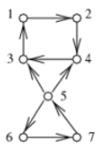Solution:

The connected component of an undirected graph is a subgraph in which any two vertices are connected to each other by paths, and is connected to no additional vertices.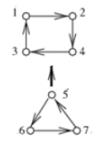QUESTION: 33

Which of the following traversal is sufficient to construct Binary search tree from traversal?

I. Preorder

II. Inorder

III. Postorder

Solution:

To construct BST either preorder or postorder is sufficient because inorder is always sorted for BST.

QUESTION: 34

Which of the following condition is user for every process by ‘Highest Response Ratio Next” scheduling to select the process with highest response ratio?

Solution:

A process which has highest Response ratio is selected to schedule next.

QUESTION: 35

Consider the following data: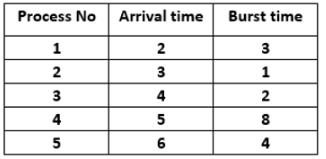Suppose round robin scheduling is applied on the given data with time quantum=2 units. It is also given that scheduling of a process or context switch will take 1 unit of time. What is the completion time and turnaround time of process P4?

Solution: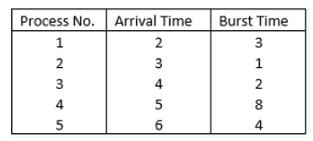Here, TQ = 2 units

Also every scheduling OT context switch will take overhead of 1ns. (Assume & represent overhead)

The Gant Chart will look like.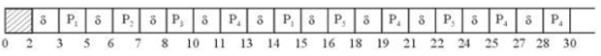Hence, completion time = 30 units

& twin around time = completion time – Arrival time

= 30 – 5

= 25 units

QUESTION: 36

What is the time complexity of the following recursive function:

int DoSomething (int n) {

if (n <= 2)

return 1;

else

return(DoSomething(floor(sqrt(n))) + n);

Solution:
QUESTION: 37

Consider the given SDT which will be executed in connection with a bottom-up parser. For the input babbba, what will be the final output?

S →aS { print “x”}

S →bS { print “y”}

S →a { print “z”}

S →b { print “z”}

Solution:

When we bottom-up parse any sequence of a’s and b’s, the input is pushed onto the stack, until the end marker isreached. At that point, the last a or b is reduced to S, and a z is printed. Then, each of the remaining a’s and b’s on the stack is replaced, along with the S on top of the stack by just an S. When we reduceusing a, we print x, and when we reduce using b, a y is printed.As a result, all input symbols, other than the last, are translated (a to x, b to y) and reversed.

A bottom-upparser begins by reading the entire input and pushing it onto the stack, so the last input symbol is at the top of the stack. Reductions occur only after the input is read, and the first must be using either production S →a or S →b.

All subsequent reductions use one of the productions S →a or S → b

QUESTION: 38

The grammar which is equivalent to -

A -> A+A/A-A/B

B -> B*B/a

After eliminating the left factoring is –

Solution:

The way of removing left factoring is-

A -> AB / AC is replaced by A -> AA'

A' -> B / C

Left factoring is only present in the first line.

So the answer is - A -> AA'/B

A' -> +A/-A

B -> B*B/a

QUESTION: 39

Consider the weighted undirected graph below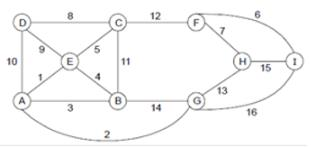Assume that edge FG is added to the minimum spanning tree. For what maximum value of FG does this new edge belong to the minimum spanning tree?

Solution:

Minimum spanning tree.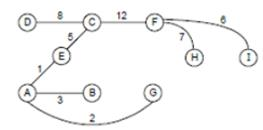If FG edge is added to the MST we can throw away largest edge in the loop (AECFGA).

FGmax=12

QUESTION: 40

Consider the following “Max-heapify” algorithm.Array has size at least n and 1in.After applying The Max-heapify noded at A[i], the result will be the subtree of A[1,….n] rooted at A[i] is a max-heapify.{ Assume that except root A[i], all its children satisfies heap property]

Max –heapify (int A[ ], int n,int i)

{ int p,m;

p = i;

while (2pn)

{

if(Y && Z)

m = 2p+1;

else m =2p;

if(A[p]<A[m])

[swap (A[p],A[m]);

p=m;

}

else

return ;

}

}

Find missing statement at Y and Z respectively to apply the heapify for subtree rooted at A[i].

Solution: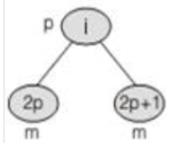if A[2p+1]> A[2p] then m=2p+1

else m= 2p

Y is : (2p+1)n

Z is : A[2p+1]>A[2p]

So, option B is correct.

QUESTION: 41

Find the type of error produced by the following program -

int main()

{

int b=10;

if(b==10)

printf("Hii user );

printf("Compiler Design");

}

Solution:

When the compiler will go in the lexical phase then ..."Hii user ); printf("…. This will be taken as a single token and when the next inverted comma will come it will go till the last of the program but will not be able to find the closing inverted comma leading to the error which will be lexical error.

QUESTION: 42

Consider n activities in the activity selection problem a1, a2, a3,…,an. For activity ai, let si be the starting time and fi be the finishing time. Then two activities ai and aj are compatible when;

Solution:

The duration of one activity should not partly or completely overlap with the duration of another activity. Their intersection should be null.

QUESTION: 43

TCP/IP was included by a _______ operating system .

Solution:

TCP/IP was included by a version of UNIX operating system.

QUESTION: 44

Consider the following operations.

(i) Reversal

(ii) Positive closure

(iii) Difference

How many of the above operations are not closed for DCFLs?

Solution:

DCFLs are not closed under Reversal, Difference and Positive closure operation.

QUESTION: 45

Match the following

List-I

(Protocol Layers)

A- Application layer

B- Network layer

List-II

Solution:

QUESTION: 46

The divergence of vector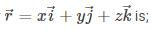Solution: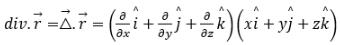= 1 + 1+ 1 = 3

QUESTION: 47

Which of the following is true regarding kernel level threads?

Solution:

a- it is false, since implementation of kernel level thread require hardware support and it is difficult as compared to user level thread.

b- It is false since kernel level threads are independent from each other. Hence, context will be larger.

c- Since each and every kernel level thread is independent of each other so there is no need of blocking of all the other threads.

d- It is true regarding kernel level threads.

QUESTION: 48

Consider a system such that the number of clock for a polling operation (including transferring to the polling routine, accessing the device and restarting) is 400 cycles, and that the processor executes with a 500 MHz clock. Determine the fraction of CPU consumed when the mouse must be polled 30 times per second.

Solution:

Check cycle for polling 30 times

= 30 400 = 12000 cycles per second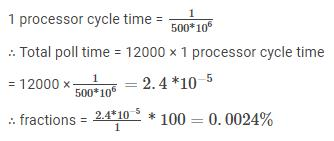QUESTION: 49

Match the following Lists:

List-I (Logic)

A-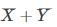B- XY

C-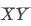List-II (Function)

1- Sum

2- NAND

3- Carry

4- NOR

Solution: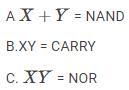QUESTION: 50

Which of the following is false?

Solution:

FD need not to be preserved in an individual table,it can be preserved by the combination of more than one table.

*Answer can only contain numeric values
QUESTION: 51

Let X be a continuous random variable with probability density function given as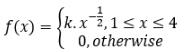Find the mean of the above distribution.

Solution:

For f(x) to be a probability density function, we need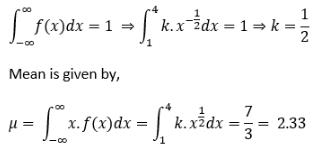*Answer can only contain numeric values
QUESTION: 52

Consider a system employing an interrupt driven I/O for a articular device that transfers data at an average of 8 KB/s on a continuous basis. Assume that interrupt processing takes about 100 micro seconds (i.e. jump to the interrupt service routine (ISR); execute it and return to the main program). Determine what fraction of processor time is consumed by this I/O device when it is interrupted for every byte.

Solution:

The device generates 8 × 1024 = 8192 bytes/sec

i.e. 1 second 8192 bytes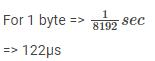Given that each interrupt consumes 100.μs

Fraction of proce ssor time consumed in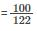(for every byte)=0.82

QUESTION: 53

Consider two processes P0 and P1 which shares a global variable ‘flag’. The value of flag is either 0 or 1 where 0 indicates “P0 is permitted to enter the critical-section” and 1 indicates for P1. Assume P0(i=0) and P1(i=1) are concurrent processes which are executing the following code with initial value of flag as 0.

while (1)

{

while( flag!=1);

<critical section>

flag = i-1;

<remainder section>

}

If P0 starts executing first, which of the following holds by the above execution.

Solution:

Mutual exclusion and bounded wait is guaranteed buy the given code.

Progress may not hold when only process is always interested to execute. process can not get consecutive execution of critical section due to after every execution turn is given to other process by making flag value to other.

*Answer can only contain numeric values
QUESTION: 54

Consider an ER model with four entity set, E1(A, B), E2(C, D), E3 (E, F), and E4 (G, H). Relation R1 is following “one to many mapping“ between E1 and E Relation R2 is following “many to one“ between E3 and E4. E1 and E4 are linked with a relation R5 following “many to many mapping”. E2 and E4 are related to each other by a one to one relationship which is R4. E1 and E3 are also related to each other by a one to one relationship (R3) but one end is showing total participation.

What is the minimum no of tables required to store the given ER Model ________

Solution:

By applying mapping Constraints, R1 can be merged with E2 with C as candidate key. Due to total participation of E3 in relation R3, (E1, R3, E3) can be merged together with E as candidate key. R4 can be merged with either E2 or E4. So, the final merged tables are

E2R1 (A, C, D)E1R3E3 (A, B, E, F)E4R4(C, G, H) R2 (E, G) R5(A, G)

Now we can merge R2 with E1R3E3, due to one to many relationship constraint. Hence, the required no of tables will be 5.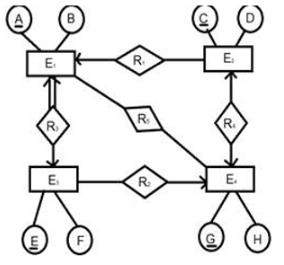QUESTION: 55

Consider the following Fibonacci recursive function used by dynamic programming.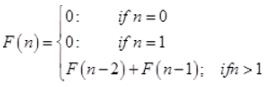Assume for every function call F(i) it checks the table first, if its value is already computed it retrieves the value from table. Otherwise it calls a recursive function call to compute its return value. Whenever a function F(i) computes first time its return value is stored in the table to avoid the redundant function calls.

How many function calls need the support of stack to complete the execution of the function F(5)?

Solution: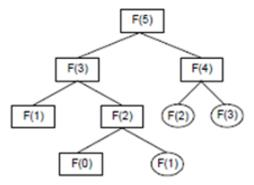F(0), F(1), F(2), F(3), F(4) and F(5) computed only one time and stored in the table. So, there are 6 functions need activation record in the stack.

QUESTION: 56

Find postfix expression for the following infix expression? Assume ↑ as the highest precedence and follow right to left associativity.

Infix: (a+b) ↑ (p+q) ↑ (r*s*t)

Solution:

The given expression is : (a+b) ↑ (p+q) ↑ (r*s*t)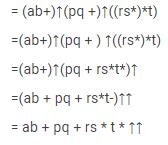QUESTION: 57

Match the following 3 address code notation with the type of address they carry in their table -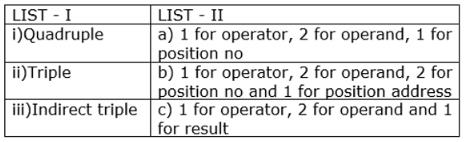Solution:

The different formats of the 3-address code are shown as below-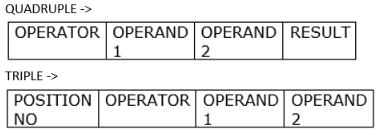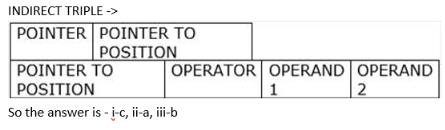QUESTION: 58

Identify the false statements:

: Separate I/O address space does not necessarily mean that I/O address lines are physically separated.

: Address decoder is an essential part of I/O interface.

Solution:

S1: Separate I/O address space does not necessarily mean that I/O address lines are physically separated from the memory address lines. A special signal on the bus indicates that the requested read or write transfer is an I/O operation.

S2: The address decoder, the data and status register, and control circuitry required to coordinate I/O transfers constitute the interface circuit (Hence true).

QUESTION: 59

Consider the following statements about Dijkstra’s algorithm.

1. Dijkstra’s algorithm produces an incorrect result only if the graph contains a negative weight cycle.

2. If a graph contains a negative weight cycle, then Dijkstra's algorithm may not terminate.

Which of the above statements is true?

Solution:

Dijkstra’s algorithm may produce incorrect result even if does not contain a negative weight cycle.

Example: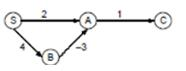The distance of C is 3 by Dijkstra's algorithm.

2. Dijkstra’s algorithm always terminates after |V| iterations.

QUESTION: 60

Match the following -

LIST- I

a) Token

b) Pattern

c) Lexeme

LIST – II

I) A rule describing the set of lexemes that can represent a particular token in the source program

II) A sequence of characters in the source program that is matched by the pattern for a token

III) A set of characters grouped together

Solution:

Token is a set of characters grouped together like literal, id, num, const, if etc.

Pattern is rule describing the set of lexemes that can represent a particular token in the source program . For example let the token be relation then its informal description of the pattern will be < or <= or = or > or >= or <> etc.

Lexeme is a sequence of characters in the source program that is matched by the pattern for a token. For example let the token be a identifier then its sample lexemes can be pi , count , a ,b etc.

QUESTION: 61

Assume a person has 10 distinct numbered balls. He wants to place these balls in 10 fixed slots of binary search tree (initially all slots are empty). In how many ways can the person place those balls in the slots of binary search tree? Each slot can occupy atmost 1 ball.

Solution:

There is only one way. The minimum value has to go to the leftmost node and the maximum value to the rightmost node. Recursively we can define for other nodes.

QUESTION: 62

Match the following: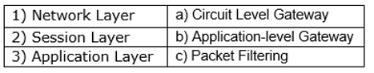Solution:

Application layer has application-level gateway. Packet filtering can be only done at Network layer as at NL we will be able to see ports and ip address of packet. Circuit level Gateway is implemented at Session Layer.

QUESTION: 63

Consider the following sequential circuit: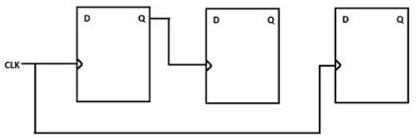If T is the propagation delay of each flip-flop, what is the maximum clock frequency which can be applied for valid functioning of the circuit?

Solution:

As we Know that,

Maximum clock frequency=1/minimum time period

Minimum Time Period = minimum time to switch from one state to another or minimum time that whole circuit can function

= T+T {because two flip-flop are cascaded}

= 2T

Hence, Maximum frequency= 1/2T

QUESTION: 64

Consider the following producer and consumer code.

# define N = 100

int mutex = 1; // Binary semaphore variable

int empty = N; // Counting semaphore variable

int full = 0; // counting semaphore variable

------------------

Void producer ( )

int item;

while (1)

item=producer-item();

down (empty);

down (mutex);

insert_item (item);

up (mutex);

up (full);

}

-----------------

Void consumer ( )

{

int item;

while (1)

{

down (mutex);

down (full);

item=consume_item();

up (mutex);

up (empty);

}

}

--------------

In the above code mutex, empty and full are semaphore shared variables and item is local to the both producer and consumer.

Inser_item (item) function will place “item” into buffer and consume_item function removes an item from the buffer. Which of the following holds by the code?

Solution:

Given code correctly implemented to solve the mutual exclusion but producer and consumer may enter the deadlock.

Deadlock may occur; if consumer executes first down (mutex) and then down (turn), consumer goes to block mode. Now producer executes down (empty) and then down (mutex), producer goes to block mode. Deadlock may occur due to the above executions when buffer is initially empty.

QUESTION: 65

The value of constant ‘a’ so that the vector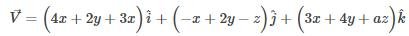is solenoidal:;

Solution:

For solenoidal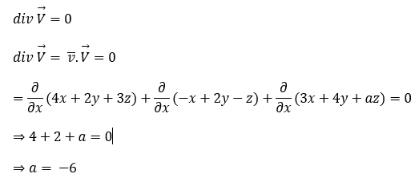Use Code STAYHOME200 and get INR 200 additional OFF Use Coupon Code

Track your progress, build streaks, highlight & save important lessons and more!

Similar ContentRelated tests